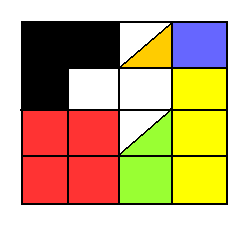# Fractions - Grade 5 Maths Questions With Solutions



Grade 5 maths multiple choice questions on fractions with answers are presented. Also Solutions and explanations are included.
Note that mixed numbers are written as follows: whole part followed by a proper fraction.
For example: $$5 \dfrac{1}{2}$$ is a mixed number meaning $$5 +\dfrac{1}{2}$$.
More resources on fractions are included.

## Questions

1. Write $$1$$ as a fraction.
1. $$\dfrac{1}{1}$$ only
2. $$\dfrac{2}{2}$$ only
3. $$\dfrac{3}{3}$$ only
4. Any fraction of the form $$\dfrac{n}{n}$$ where $$n$$ is a whole number
Solution
2. Write 5 as a reduced fraction.
1. $$\dfrac{5}{5}$$
2. $$\dfrac{1}{5}$$
3. $$\dfrac{5}{1}$$
4. $$\dfrac{1}{1}$$
Solution
3. $\dfrac{1}{4} + \dfrac{2}{4} =$
1. $$\dfrac{3}{4}$$
2. $$\dfrac{3}{8}$$
3. $$\dfrac{7}{8}$$
4. $$3$$
Solution
4. $\dfrac{4}{7} - \dfrac{2}{7} =$
1. $$\dfrac{2}{14}$$
2. $$\dfrac{6}{7}$$
3. $$\dfrac{2}{7}$$
4. $$\dfrac{4}{7}$$
Solution
5. $\dfrac{1}{5} + \dfrac{2}{3} =$
1. $$\dfrac{3}{8}$$
2. $$\dfrac{2}{15}$$
3. $$\dfrac{1}{8}$$
4. $$\dfrac{13}{15}$$
Solution
6. $3 \dfrac{1}{2} + 5 \dfrac{1}{3}$
1. $$8$$
2. $$8 \dfrac{2}{5}$$
3. $$8 \dfrac{5}{6}$$
4. $$\dfrac{2}{5}$$
Solution

7. It takes Julia $$\dfrac{1}{2}$$ hour to wash, comb her hair and put on her clothes, and $$\dfrac{1}{4}$$ hour to have her breakfast. How much time does it take Julia to be ready for school?
1. $$\dfrac{3}{4}$$ hour
2. 1 hour
3. $$\dfrac{2}{4}$$ hour
4. 1 and $$\dfrac{1}{4}$$ hours
Solution

8. Which two fractions are equivalent?
1. $$\dfrac{5}{2}$$ and $$\dfrac{2}{5}$$
2. $$\dfrac{4}{3}$$ and $$\dfrac{8}{6}$$
3. $$\dfrac{1}{4}$$ and $$\dfrac{2}{4}$$
4. $$\dfrac{2}{3}$$ and $$\dfrac{1}{3}$$
Solution
9. $5 \dfrac{2}{3} - 3 \dfrac{1}{2} =$
1. $$2$$
2. $$1 \dfrac{2}{5}$$
3. $$2 \dfrac{7}{6}$$
4. $$2 \dfrac{1}{6}$$
Solution

10. Billy ate 1 and $$\dfrac{1}{4}$$ of pizzas and John ate 1 and $$\dfrac{2}{3}$$ pizzas. How much more pizza did John eat than Billy?
1. $$\dfrac{2}{3}$$
2. $$\dfrac{5}{12}$$
3. $$\dfrac{1}{4}$$
4. $$\dfrac{7}{12}$$
Solution
11. $\dfrac{5}{2} \div \dfrac{3}{4}$
1. $$\dfrac{10}{3}$$
2. $$\dfrac{10}{8}$$
3. $$\dfrac{13}{4}$$
4. $$1$$
Solution

12. $5 \div \dfrac{1}{7}$
1. $$\dfrac{5}{7}$$
2. $$\dfrac{6}{7}$$
3. $$\dfrac{1}{35}$$
4. $$35$$
Solution-11
13. $\dfrac{2}{5} \times \dfrac{3}{7}$
1. $$\dfrac{14}{15}$$
2. $$\dfrac{6}{35}$$
3. $$\dfrac{35}{6}$$
4. $$\dfrac{15}{14}$$
Solution

14. To have $$a + 1 \dfrac {3}{4} = 2$$ , $$a$$ must be equal to
1. $$1$$
2. $$\dfrac{3}{4}$$
3. $$\dfrac{1}{2}$$
4. $$\dfrac{1}{4}$$
Solution

15. What fraction or mixed number is the shaded part?.

1. $$\dfrac{3}{4}$$
2. $$\dfrac{6}{4}$$
3. $$2 \dfrac{3}{4}$$
4. $$1 \dfrac{3}{4}$$
Solution

16. True or false $2 \dfrac{1}{2} = 2 \times \dfrac{1}{2}$ Solution

17. Tina works 15 hours a week (Monday to Friday). Last week she worked 3 and 1/2 hours on Monday, 4 hours on Tuesday, 2 and 1/6 hours on Wednesday and 1 and 1/2 hours on Thursday. How many hours did she work on Friday?
1. $$4$$
2. $$\dfrac{5}{6}$$
3. $$3 \dfrac{5}{6}$$
4. $$2 \dfrac{5}{6}$$
Solution

18. Which point on the number line represents $$1 \dfrac{7}{10}$$?.

1. s
2. R
3. W
4. K
Solution

19. Write $$2 \dfrac{1}{3}$$ as an improper fraction.
1. $$\dfrac{2}{3}$$
2. $$\dfrac{7}{3}$$
3. $$\dfrac{1}{3}$$
4. $$\dfrac{3}{3}$$
Solution

20. Write the fraction $$\dfrac{31}{8}$$ as a mixed number.
1. $$4$$
2. $$4 \dfrac{7}{8}$$
3. $$3 \dfrac{1}{8}$$
4. $$3 \dfrac{7}{8}$$
Solution

21. $3 \times \dfrac{1}{4} =$
1. $$3 \dfrac{1}{4}$$
2. $$\dfrac{1}{4} + \dfrac{1}{4} + \dfrac{1}{4}$$
3. $$\dfrac{1}{4}$$
4. $$12$$
Solution

22. $3 \dfrac{1}{4} =$
1. $$3 \times \dfrac{1}{4}$$
2. $$\dfrac{3}{4}$$
3. $$3 + \dfrac{1}{4}$$
4. $$\dfrac{4}{3}$$
Solution

23. True or false $\dfrac{2}{5} \gt \dfrac{3}{8}$ Solution

24. Order from least to greatest the fractions $\dfrac{3}{5} \; , \; \dfrac{7}{6} \; , \; \dfrac{1}{3} \; , \; \dfrac{4}{9}$
1. $$\dfrac{1}{3} \; , \; \dfrac{4}{9} \; , \; \dfrac{3}{5} \; , \; \dfrac{7}{6}$$
2. $$\dfrac{4}{9} \; , \; \dfrac{1}{3} \; , \; \dfrac{3}{5} \; , \; \dfrac{7}{6}$$
3. $$\dfrac{1}{3} \; , \; \dfrac{4}{9} \; , \; \dfrac{7}{6} \; , \; \dfrac{3}{5}$$
4. $$\dfrac{1}{3} \; , \; \dfrac{3}{5} \; , \; \dfrac{4}{9} \; , \; \dfrac{7}{6}$$
Solution

25. Write $$\dfrac{2}{3}$$ of $$4$$ as a mixed number.
1. $$4 \dfrac{2}{3}$$
2. $$1 \dfrac{2}{3}$$
3. $$2 \dfrac{2}{3}$$
4. $$\dfrac{8}{3}$$
Solution

26. How many minutes are there in $$\dfrac{2}{3}$$ of an hour?
1. 40 minutes
2. 60 minutes
3. 20 minutes
4. 100 minutes
Solution
27. In the figure below, a large square was divided into 16 smaller squares of equal sides.
What fraction of the large square is red?
What fraction of the large square is blue?
What fraction of the large square is orange?
What fraction of the large square is green?
What fraction of the large square is black?
What fraction of the large square is yellow?.

1. red: $$\dfrac{1}{4}$$ , blue: $$\dfrac{1}{16}$$ , orange: $$\dfrac{1}{16}$$, green: $$\dfrac{3}{16}$$, black: $$\dfrac{3}{16}$$, yellow: $$\dfrac{3}{16}$$
2. red: $$\dfrac{4}{4}$$ , blue: $$\dfrac{1}{16}$$ , orange: $$\dfrac{1}{16}$$ , green:$$\dfrac{3}{32}$$ , black: $$\dfrac{3}{16}$$ , yellow: $$\dfrac{3}{16}$$
3. red: $$\dfrac{1}{4}$$ , blue: $$\dfrac{1}{16}$$ , orange: $$\dfrac{1}{16}$$ , green: $$\dfrac{3}{16}$$ , black: $$\dfrac{3}{16}$$ , yellow: $$\dfrac{3}{16}$$
4. red: $$\dfrac{1}{4}$$ , blue: $$\dfrac{1}{16}$$ , orange: $$\dfrac{1}{32}$$ , green: $$\dfrac{3}{32}$$ , black: $$\dfrac{3}{16}$$ , yellow: $$\dfrac{3}{16}$$
Solution

1. D
2. C
3. A
4. C
5. D
6. C
7. A
8. B
9. D
10. B
11. A
12. D
13. B
14. D
15. C
16. false
17. C
18. C
19. B
20. D
21. B
22. C
23. True
24. A
25. C
26. A
27. D